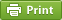Werner's Blog — Opinion, Analysis, Commentary
Fictitious econometric precision

When economists report results of empirical analysis, they often purport to show great accuracy. An estimate might be reported as 1.234567 rather than "about 1". My University of Victoria colleague Dave Giles raised this as a question in his December 15, 2011 blog about Reported "Accuracy" for Rregression Results. How many significant digits should we report in estimation results? Does it depend on the quality and accuracy of the raw data, or the statistical properties of what is estimated?

This blog is an invitation to econometricians to set the record straight on reporting meaningful results. What should be considered "best practice"? My musings below are probably a rather crude attempt to get to the point, and perhaps there are better methods available than the procedure I describe below. Your comments and feedback will be greatly appreciated.

Econometric work involves reporting lots of estimates along with their standard errors (or standard scores and p-values). Call it laziness, but our tendency is to report the numbers the way they come out of the statistical software, such as Stata, R, or SAS. Statistical software is set up to report a fixed number of digits regardless of whether extra digits are meaningful or not. This can sometimes lead to bizarre outcomes. More than once I have seen a near-zero estimate reported as 0.000 when in fact it may have been 0.0001. Rounding it down to zero could make sense, but that depends entirely on the precision of the estimate.

What is commonly referred to as precision is, in the Bayesian sense, the inverse of variance. At the extremes we have a perfectly precise number (which in turn has zero variance) and a perfectly imprecise number (which has infinite variance). When we look at a conventional estimate, let's say 0.9173582, we should ask ourselves about the confidence we have in each digit of this estimate. Are all eight digits significant? Is the last digit "2" meaningful, or the last "582"? In some disciplines, such as physics, precision matters a lot to confirm a discovery. Physics employs a 5-sigma standard; a discovery is only confirmed as such if the probability of it being a statistical fluke is less than 1 in 3.5 million. In statistical parlance, a five-sigma standard ensures that the null hypothesis (of no discovery) is rejected erroneously with a probability of less than $$3\cdot10^{-7}$$. Some branches of physics demand an even higher 10-sigma standard. Economics is nothing like that. Economists are usually satisfied with a 1.96-sigma standard, which corresponds to a 95% level of confidence. It is important to stress that our confidence is in the sampling procedure that generated the estimate, not the estimate itself. With a 95% level of confidence we can infer that there are 95 chances in 100 that the sampling procedure that generated the data will produce a result within the vicinity of 1.96 standard deviations of the original estimate.

When it comes to testing a null hypothesis, we employ a standard score $$z$$ defined as $z=\frac{x-\mu}{s_x}$ where $$x$$ is the estimate, $$\mu$$ is the true mean, and $$s_x$$ is the standard error. The standard score is then compared against the appropriate statistical distribution, and this determines whether we accept or reject the null hypothesis. Let us assume that our estimate 0.9173582 was reported with a standard error of 0.1234567. The default null hypothesis investigates whether our estimate is different from zero. Then our z-score is 7.431, which turns out to be highly significant statistically when we employ a normal distribution for testing. When we test whether our estimate is different from one, though, we find a z-score of –0.669, which is not at all statistically significant.

We can now look at the statistical significance of our estimate rounded up or down, digit by digit, from right to left, as shown in the table below.

Log10 Precision True Mean |z|-score p-value
–7   0.0000001   0.9173582 <0.001 <0.0001
–6   0.000001   0.917358 <0.001 <0.0001
–5   0.00001   0.91736 <0.001 <0.0001
–4   0.0001   0.9174 <0.001 0.0003
–3   0.001   0.917 0.0029 0.0023
–2   0.01   0.92 0.0214 0.0171
–1   0.1   0.9 0.1406 0.1118
0   1.   1. 0.6694 0.4968

Take the line with a precision of 0.0001 and a true mean of 0.9174. With respect to that number, our estimate has a tiny absolute z-score and its corresponding p-value is virtually nil. We can be sure that we haven't lost any significance here. Moving to the next line, rounding to 0.917 implies a p-value of 0.0023. The margin of error due to rounding is still tiny. Rounding to 0.92 increases the p-value to 1.71%. But when we round to 0.9 we clearly lose precision. The p-value rises to 0.11. So which rounding should we choose? As a rule of thumb, rounding should not introduce an error more than 5%, and probably less than 1% would be acceptable for most practical purposes. In other words, 0.92 is perfectly fine (the p-value is less than 5%) and 0.917 is reasonably exact (the p-vale is less than 1%).

Different estimates have different precision, and thus the rounding mechanism should be applied individually to each estimate. Below is a short code fragment in SAS that shows how to obtain a "reasonably rounded" estimate. The SAS function "round(a,b)" rounds a number "a" to the precision "b" (e.g., 0.001). The function "probnorm(z)" returns the probability that an observation from the standard normal distribution is less than or equal to the score "z".

*-------------------------------------------------------------+ | Regression Estimate Rounding Pseudo Code (SAS) | +-------------------------------------------------------------; %MACRO ROUNDED(estimate,stderr); _r=floor(log10(&estimate))-1; _e=1; do while(_e>1E-2); _x=round(&estimate,10**_r); _e=2*probnorm(abs((&estimate-_x)/&stderr))-1; _r=_r-1; end; %MEND;
Posted on Saturday, October 31, 2015 at 09:45 — #EconometricsRecent Blog Entries

Topics

Months

Subscribe to RSS feed© 2023  Prof. Werner Antweiler, University of British Columbia.